# JP5609585B2 - PLL circuit, PLL circuit error compensation method, and communication apparatus - Google Patents

PLL circuit, PLL circuit error compensation method, and communication apparatus Download PDF

## Info

Publication number
JP5609585B2
JP5609585B2 JP2010262781A JP2010262781A JP5609585B2 JP 5609585 B2 JP5609585 B2 JP 5609585B2 JP 2010262781 A JP2010262781 A JP 2010262781A JP 2010262781 A JP2010262781 A JP 2010262781A JP 5609585 B2 JP5609585 B2 JP 5609585B2
Authority
JP
Japan
Prior art keywords
clock
circuit
unit
output
fractional
Prior art date
Legal status (The legal status is an assumption and is not a legal conclusion. Google has not performed a legal analysis and makes no representation as to the accuracy of the status listed.)
Expired - Fee Related
Application number
JP2010262781A
Other languages
Japanese (ja)
Other versions
JP2012114736A5 (en
JP2012114736A (en
Inventor

Original Assignee
ソニー株式会社
Priority date (The priority date is an assumption and is not a legal conclusion. Google has not performed a legal analysis and makes no representation as to the accuracy of the date listed.)
Filing date
Publication date
Application filed by ソニー株式会社 filed Critical ソニー株式会社
Priority to JP2010262781A priority Critical patent/JP5609585B2/en
Publication of JP2012114736A publication Critical patent/JP2012114736A/en
Publication of JP2012114736A5 publication Critical patent/JP2012114736A5/ja
Application granted granted Critical
Publication of JP5609585B2 publication Critical patent/JP5609585B2/en
Expired - Fee Related legal-status Critical Current
Anticipated expiration legal-status Critical

• 229920000729 poly(L-lysine) polymers Polymers 0.000 title claims description 19
• 244000241601 filaree Species 0.000 claims description 228
• 238000004364 calculation methods Methods 0.000 claims description 71
• 241001442055 Vipera berus Species 0.000 claims description 53
• 230000001186 cumulative Effects 0.000 claims description 52
• 230000000630 rising Effects 0.000 claims description 43
• 238000006243 chemical reactions Methods 0.000 description 19
• 230000000737 periodic Effects 0.000 description 17
• 238000010586 diagrams Methods 0.000 description 15
• 238000000034 methods Methods 0.000 description 11
• 238000004088 simulation Methods 0.000 description 9
• 230000004913 activation Effects 0.000 description 6
• 230000005540 biological transmission Effects 0.000 description 6
• 101710074301 ALDH2C4 Proteins 0.000 description 3
• 101710003274 APEX1 Proteins 0.000 description 3
• 101710005470 CYP83A1 Proteins 0.000 description 3
• 101710003569 REF2 Proteins 0.000 description 3
• 102100008882 Tissue factor pathway inhibitor 2 Human genes 0.000 description 3
• 230000000875 corresponding Effects 0.000 description 3
• 101710068105 tissue-factor-pathway inhibitor 2 Proteins 0.000 description 3
• 238000009825 accumulation Methods 0.000 description 2
• 230000003213 activating Effects 0.000 description 2
• 230000004048 modification Effects 0.000 description 2
• 238000006011 modification reactions Methods 0.000 description 2
• 239000004065 semiconductors Substances 0.000 description 2
• 239000000969 carriers Substances 0.000 description 1
• 238000004590 computer program Methods 0.000 description 1
• 230000003111 delayed Effects 0.000 description 1
• 230000000694 effects Effects 0.000 description 1
• 238000003780 insertion Methods 0.000 description 1
• 238000004519 manufacturing process Methods 0.000 description 1
• 230000001960 triggered Effects 0.000 description 1

## Images

•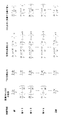•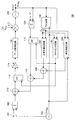•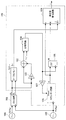•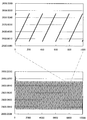•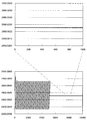•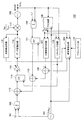•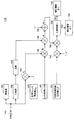•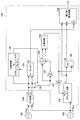•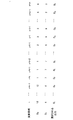•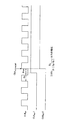•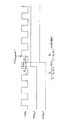•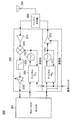•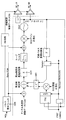•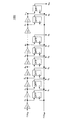•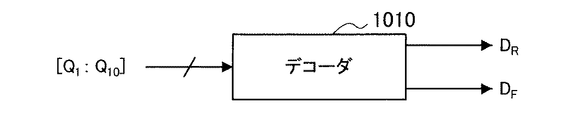•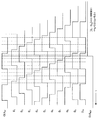•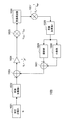•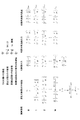## Classifications

• HELECTRICITY
• H03BASIC ELECTRONIC CIRCUITRY
• H03LAUTOMATIC CONTROL, STARTING, SYNCHRONISATION, OR STABILISATION OF GENERATORS OF ELECTRONIC OSCILLATIONS OR PULSES
• H03L7/00Automatic control of frequency or phase; Synchronisation
• H03L7/06Automatic control of frequency or phase; Synchronisation using a reference signal applied to a frequency- or phase-locked loop
• H03L7/16Indirect frequency synthesis, i.e. generating a desired one of a number of predetermined frequencies using a frequency- or phase-locked loop
• HELECTRICITY
• H03BASIC ELECTRONIC CIRCUITRY
• H03LAUTOMATIC CONTROL, STARTING, SYNCHRONISATION, OR STABILISATION OF GENERATORS OF ELECTRONIC OSCILLATIONS OR PULSES
• H03L2207/00Indexing scheme relating to automatic control of frequency or phase and to synchronisation
• H03L2207/50All digital phase-locked loop

## Description

The present invention relates to a PLL circuit, an error compensation method for a PLL circuit, and a communication apparatus.

In the wireless communication terminal, a PLL (Phase Locked Loop) circuit is used to lock the carrier frequency to an accurate frequency. In recent years, with the miniaturization of semiconductor processes, a configuration in which a voltage controlled oscillator (Voltage Controlled Oscillator, VCO) controlled by an analog voltage is replaced with a digitally controlled oscillator (Digital Controlled Oscillator, DCO) is drawing attention.

A conventional PLL circuit using a VCO compares a phase difference between two clocks of a reference clock and a clock obtained by dividing the VCO output by a phase comparator. Here, as a general phase comparator, a circuit that converts the phase difference into three-state pulse widths of up, down, and up + down is used, and this pulse is used to control the current source of the charge pump circuit. The output current is converted into a voltage by a loop filter, and the VCO is controlled.

On the other hand, as shown in FIG. 11, an example of an All-Digital PLL circuit using a DCO that has been attracting attention in recent years is a time-to-digital converter (TDC) circuit in which a fractional component of the cumulative number of clocks corresponding to a phase difference is obtained. The Integrer component is converted into a digital value by an accumulator circuit, and a digital value corresponding to the detected phase difference is fed back by various methods to digitally control the DCO.

R.B.Staszewskiet al., "All-Digital Phase-Domain TX FrequencySynthesizer for Bluetooth Radios in 0.13um CMOS, ISSCC2004 Digest

However, when a small division ratio is set in a PLL using a TDC circuit, there is a concern that a periodic error may occur due to the resolution of the TDC circuit. The periodic error caused by the resolution of the TDC circuit has a problem of generating spurious components.

Therefore, the present invention has been made in view of the above problems, and an object of the present invention is to compensate for periodic errors caused by the finite resolution of a TDC circuit used in a PLL circuit having a digitally controlled oscillator. It is an object of the present invention to provide a new and improved PLL circuit, an error compensation method for a PLL circuit, and a communication device that can be used.

In order to solve the above-described problem, according to an aspect of the present invention, an accumulated clock number detection unit that detects the accumulated clock number of the oscillation circuit as a digital value, and a digital value of a decimal part of the accumulated clock number of the oscillation circuit A period detector for detecting periodicity with reference to the first reference clock, a digital value of one period of the output clock of the oscillation circuit, and one period of periodicity possessed by the fractional part of the accumulated clock number of the oscillation circuit A correction value calculating unit that calculates a correction value from the digital value of the first and second reference clock numbers from a starting point of each periodicity of the fractional part of the accumulated clock number of the oscillation circuit; and There is provided a PLL circuit comprising: an addition unit that adds the correction value to the decimal part of the cumulative clock number in the period of the first reference clock from the start point of each periodicity period.

The accumulated clock number detection unit may include a TDC circuit that detects a decimal part of the accumulated clock number of the oscillation circuit.

The switching unit further switches whether or not the correction value is added by the adding unit, and the switching unit is a one-cycle digital signal having a periodicity included in the frequency division ratio, the resolution of the TDC circuit, and the fractional part of the accumulated clock number. Based on the relationship between the value and a constant that controls the loop band, switching of whether or not the correction value is added by the adding unit may be controlled.

A convergence detection unit that detects a convergence state of the PLL, and the convergence detection unit controls switching of whether or not the correction value is added by the addition unit after reaching a set arbitrary convergence state; Also good.

A retiming unit for retiming the first reference clock with an output clock of the oscillation circuit or a clock obtained by dividing the output clock; and the accumulated clock number detecting unit is configured so that the retiming unit A clock obtained by retiming the reference clock may be used as the second reference clock, and the output of the integer part of the cumulative number of clocks of the oscillation circuit may be held at the timing of the rising edge of the second reference clock. .

When the retiming unit uses a rising edge as an edge for retiming, the cumulative clock number detecting unit divides the rising edge of the first reference clock from the output clock of the oscillation circuit or the output clock. You may make it detect the periodicity of the digital value of the time difference between the rising edges of the rounded clock.

When the retiming unit uses a falling edge as an edge for retiming, the accumulated clock number detecting unit uses the rising edge of the first reference clock and the output clock of the oscillation circuit or the output clock. You may make it detect the periodicity of the digital value of the time difference between the falling edges of the divided clock.

From the value obtained by cumulatively adding the frequency division ratio represented by the digital value for each first reference clock, the cumulative clock number of the oscillation circuit held at the timing of the rising edge of the second reference clock. There may be further provided a phase comparator that performs an operation of subtracting the digital value of the integer part and the digital value of the decimal part of the accumulated clock number of the oscillation circuit.

When the retiming unit uses a rising edge as an edge for retiming, the phase comparator serves as the fractional part of the accumulated clock of the oscillation circuit, and outputs the first of the outputs of the accumulated clock number detecting unit. A fractional part calculated from the digital value of the time difference between the rising edge of the reference clock and the output clock of the oscillation circuit or the rising edge of the clock obtained by dividing the output clock may be used.

When the retiming unit uses a falling edge as an edge to be retimed, the phase comparator serves as a fractional part of the accumulated clock of the oscillation circuit, and outputs the first of the outputs of the accumulated clock number detecting unit. A decimal part calculated from the digital value of the time difference between the rising edge of one reference clock and the output clock of the oscillation circuit or the falling edge of the clock obtained by dividing the output clock may be used.

In order to solve the above problem, according to another aspect of the present invention, an accumulated clock number detecting step for detecting the accumulated clock number of the oscillation circuit as a digital value, and a decimal part of the accumulated clock number of the oscillation circuit are provided. A period detection step for detecting a periodicity of a digital value with reference to a first reference clock, a digital value of one period of the output clock of the oscillation circuit, and a periodicity of a fractional part of the accumulated clock number of the oscillation circuit A correction value calculating step of calculating a correction value from the digital value of one cycle of the first and second counts of the first reference clock from the starting point of each periodicity of the fractional part of the cumulative number of clocks of the oscillation circuit And an addition step of adding the correction value to the fractional part of the cumulative clock number in the period of the first reference clock from the start point of each period of the periodicity, Error compensation method of LL circuit is provided.

Moreover, in order to solve the said subject, according to another viewpoint of this invention, a communication apparatus provided with the said PLL circuit is provided.

As described above, according to the present invention, a novel and improved PLL circuit capable of compensating for a periodic error caused by the finite resolution of a TDC circuit used in a PLL circuit having a digitally controlled oscillator, PLL A circuit error compensation method and a communication apparatus can be provided.

It is explanatory drawing shown about calculation of the correction value at the time of guaranteeing the periodicity error which generate | occur | produces with the finite resolution of the TDC circuit used for the PLL circuit with a digital control oscillator. It is explanatory drawing which shows the structure of the PLL circuit 100 concerning the 1st Embodiment of this invention. It is explanatory drawing which shows the structure of the correction value calculation part 109 contained in the PLL circuit 100 concerning the 1st Embodiment of this invention. It is explanatory drawing which shows the simulation result which does not consider the correction value by the PLL circuit 100 concerning the 1st Embodiment of this invention in a graph. It is explanatory drawing which shows the simulation result which considered the correction value by the PLL circuit 100 concerning the 1st Embodiment of this invention in the graph. It is explanatory drawing which shows the structure of PLL circuit 100 'concerning the 2nd Embodiment of this invention. It is explanatory drawing which shows the structure of the activation part 119 contained in PLL circuit 100 'concerning the 2nd Embodiment of this invention. It is explanatory drawing which shows the structure of the correction value calculation part 109 'contained in the PLL circuit contained in the 3rd Embodiment of this invention. FIG. 3 is an explanatory diagram showing which of the two outputs of the TDC circuit 106 is controlled by the switch 130 to be input to the first delay circuit 123. It is explanatory drawing which shows the phase comparison process by the correction value calculation part 109 'contained in the PLL circuit concerning the 3rd Embodiment of this invention. It is explanatory drawing which shows the phase comparison process by the correction value calculation part 109 'contained in the PLL circuit concerning the 3rd Embodiment of this invention. It is explanatory drawing explaining the structure of the radio | wireless terminal apparatus 200 provided with the PLL circuit concerning each embodiment of this invention. It is explanatory drawing which shows the example of the All-Digital PLL circuit using DCO. It is explanatory drawing which shows the structure of a general TDC circuit. It is explanatory drawing which shows the structure of a general TDC circuit. It is explanatory drawing which shows the process of the digital conversion in a general TDC circuit with a timing chart. It is explanatory drawing which shows the behavior model of generation | occurrence | production of the periodicity error resulting from the resolution of a TDC circuit. It is explanatory drawing which shows what calculated the value in each block based on the behavior model shown in FIG.

Exemplary embodiments of the present invention will be described below in detail with reference to the accompanying drawings. In addition, in this specification and drawing, about the component which has the substantially same function structure, duplication description is abbreviate | omitted by attaching | subjecting the same code | symbol.

The description will be made in the following order.
<1. Problems of conventional PLL circuit>
<2. First Embodiment>
[2-1. Calculation of correction value]
[2-2. Configuration of PLL circuit]
[2-3. Configuration of correction value calculation unit]
[2-4. simulation result]
<3. Second Embodiment>
[3-1. Configuration of PLL circuit]
[3-2. Functional configuration of the activate circuit]
<4. Third Embodiment>
[4-1. Configuration of correction value calculation unit]
[4-2. Phase comparison process]
<5. Wireless terminal device provided with PLL circuit>
<6. Summary>

<1. Problems of conventional PLL circuit>
The resolution of the TDC circuit used for detecting the fractional part of the accumulated clock number of the output clock of the digitally controlled oscillator (DCO) has a certain finite resolution depending on the semiconductor process. 12 and 13 are explanatory diagrams showing a configuration of a general TDC circuit. Hereinafter, the configuration of a general TDC circuit will be described with reference to FIGS. 12 and 13.

In a general TDC circuit 1000, elements having a minute delay Δt, such as an inverter circuit, are connected to each other, and an oscillation frequency clock is input to this delay element. At the rising edge of the reference clock, the output of each delay element is held, and the held data is decoded by the decoder 1010. Thereby, the time difference between the rising edge of the reference clock and the rising edge of the oscillation frequency clock or the time difference between the falling edges can be digitally converted.

FIG. 14 is an explanatory diagram showing a digital conversion process in a general TDC circuit in a timing chart. Hereinafter, digital conversion processing in the TDC circuit will be described with reference to the timing chart of FIG. When CLK RF is input to the TDC circuit 1000 in FIG. 12, the outputs D 1 to D 10 of the delay elements have waveforms delayed by Δt. When these waveforms are held at the rising edge of CLK RF, the digital data [Q 1 : Q 10 ] composed of the outputs of the flip-flops Q 1 to Q 10 becomes “1110000011”. The decoder circuit 1010 counts this data from the left, and a point where the data changes from 1 to 0 is t R , and a point where the data changes from 0 to 1 is t F. That is, in the example of FIG. 14, t R and t F are digitally converted to t R = 3Δt and t F = 8Δt, respectively.

In a PLL using a TDC circuit, when a small frequency division ratio is set, there is a concern about the occurrence of periodic errors due to the resolution of the TDC circuit. A behavior model used to explain this phenomenon is shown in FIG. FIG. 16 shows a value calculated for each block based on this behavior model. Here, the value of the fractional part of the frequency division ratio is limited to a very small condition, and only the influence of the fractional part is considered. First, assume that the initial value of the decimal part of the cumulative adder 1 in the calculation cycle 0 is (Δt / T) · (α / M), and the initial value of the decimal part of the cumulative adder 2 is Δt / T. Here, Δt is the time resolution of the TDC circuit, T is the period of the oscillation frequency, M is the number of divisions with respect to Δt / T of the decimal part of the set division ratio, and α is an arbitrary integer value. Therefore, the adder output in the calculation cycle 0 is (Δt / T) · {(α / M) −1}. Further, when the variable gain circuit (G 1 = 1/2 α ) and the DCO gain (G DCO = f ref ) are taken into account, the error of the oscillation frequency becomes a value shown in the following (1).

Next, the decimal part of the cumulative adder 2 in the calculation cycle 1 is obtained by multiplying the value Δt / T in the calculation cycle 0 by G 2 (= 1 / f ref ) by the desired oscillation frequency. Δt / T) · (1 / M) is added, and further, a value obtained by multiplying the oscillation frequency error in the calculation cycle 0 expressed by the above (1) by G 2 is added. The reason for multiplying G 2 in multiplier addition processing in the accumulator 2, is to be done for each reference clock f ref. Therefore, the decimal part of the cumulative adder 2 in the calculation cycle 1 is a value represented by the following (2).

Thereafter, when this calculation is repeated, the decimal part of the cumulative adder 2 in the calculation cycle M becomes a value represented by the following (3).

Here, in (3) above, α = (M + 1) / 2 is obtained when the condition in which the parentheses are 0 is obtained. The condition that the parentheses in the decimal part of the cumulative adder 2 become zero is equivalent to the average frequency error over M periods becoming zero. That is, the average value of the oscillation frequency of the M period is controlled to the set frequency. Here, when the error of the adder output in the calculation cycle M is calculated, the error of the adder output in the calculation cycle M becomes a value represented by the following (4).

Thereby, it can be seen that the error of the adder output in the calculation cycle M is the same as the error of the adder output in the calculation cycle 0. From the above, it can be understood from the calculation that this operation is repeated in the period M. This periodic error generates a spurious component f ref / M.

Thus, in each embodiment of the present invention described below, a PLL circuit capable of compensating for a periodic error caused by a finite resolution of a TDC circuit used in a PLL circuit having a digitally controlled oscillator will be described.

<2. First Embodiment>
[2-1. Calculation of correction value]
FIG. 1 is an explanatory diagram showing calculation of a correction value when guaranteeing a periodic error caused by a finite resolution of a TDC circuit used in a PLL circuit having a digitally controlled oscillator according to the first embodiment of the present invention. It is. Hereinafter, calculation of a correction value when guaranteeing a periodic error will be described with reference to FIG.

Here, the initial value of the decimal part of the cumulative number of clocks of the output clock of the oscillation circuit in the operation cycle M is 2Δt / T, and the initial value of the decimal part of the cumulative addition value of the set division ratio is (Δt / T) · {(Α + M) / M)}. Further, x is a correction value to be added to the fractional part of the cumulative clock number of the output clock of the oscillation circuit. The output of the phase comparator in the calculation cycle M is (5) below.

Here, when obtaining the correction value x M of the output of the phase comparator in the calculation cycle M 0 becomes (Δt / T) · {( α / M) -1)}. Similarly, the correction value in each calculation cycle is obtained as follows.

That is, as can be seen from the above, it can be seen that the correction value is repeated in the period M. When the start point of the periodicity of this calculation cycle is set to 0 and the correction value xk in an arbitrary calculation cycle k is expressed, the following formula 1 is obtained.

Thus, the compensation in each calculation cycle k, by Toko continues by adding the correction value x k to the value of the fractional part of the accumulated clock count of an oscillator circuit is calculated by using the TDC circuit output, the output of the phase comparator to 0 It becomes possible to continue doing.

The calculation of the correction value when guaranteeing the periodic error generated by the finite resolution of the TDC circuit used in the PLL circuit having the digitally controlled oscillator has been described above. Next, the configuration of the PLL circuit according to the first embodiment of the present invention will be described.

[2-2. Configuration of PLL circuit]
FIG. 2 is an explanatory diagram showing the configuration of the PLL circuit 100 according to the first embodiment of the present invention. The configuration of the PLL circuit 100 according to the first embodiment of the present invention will be described below with reference to FIG.

As shown in FIG. 2, the PLL circuit 100 according to the first embodiment of the present invention includes a first cumulative adder 102, a first reference clock 103, a second cumulative adder 104, and an oscillation. Circuit 105, TDC circuit 106, decimal part calculation circuit 107, first adder 108, correction value calculation unit 109, retiming circuit 110, first flip-flop 111, and second adder 112, a third adder 113, a second flip-flop 114, a variable gain circuit 115, a data conversion circuit 116, and a multiplier 117.

The first cumulative adder 102 cumulatively adds the digitally divided set frequency division ratio “N” 101 for each rising edge of the first reference clock 103. The second cumulative adder 104 cumulatively adds the value “1” for each rising edge of the output clock CLK RF of the oscillation circuit 105. That is, the second cumulative adder 104 is a circuit that counts the number of clocks of the output clock CLK RF of the oscillation circuit 105.

The TDC circuit 106 includes a time difference between the rising edge of the output clock CLK RF of the oscillation circuit 105 and the rising edge of the first reference clock 103, and the falling edge of the output clock CLK RF of the oscillation circuit 105 and the first reference clock 103. This is a circuit for digitally converting the time difference between the rising edges. Fractional part calculating circuit 107, the D R and D F digitally converted by the TDC circuit 106, according to Equation 2 below, calculates the fractional part. Note that DT may be configured such that values calculated in each cycle of the first reference clock 103 are averaged for a certain period, and the averaged value is constantly updated and used for calculation of Equation 2.

This decimal part is a decimal part of the cumulative number of clocks of the output clock CLK RF of the oscillation circuit 105. The first adder 108 adds the correction value calculated by the correction value calculation unit 109 to the decimal part calculated by the above (Formula 2). The retiming circuit 110 generates a second reference clock obtained by retiming the first reference clock 103 at the rising edge of the output clock CLK RF of the oscillation circuit 105.

The first flip-flop 111 holds the output of the second cumulative adder 104 at the rising edge of the second reference clock that has been retimed. This is equivalent to calculating an integer value obtained by rounding up the decimal part when displaying the cumulative clock number of the output clock CLK RF of the oscillation circuit 105 as a decimal point.

The second adder 112 subtracts, from the output of the first flip-flop 111, a value obtained by adding the correction value calculated by the correction value calculation unit 109 to the decimal part calculated by the above (Formula 2). The output of the second adder 112 is a digital value of the cumulative number of clocks indicated by the decimal point of the output clock CLK RF of the oscillation circuit 105. Therefore, the third adder 113 displays the decimal point of the output clock CLK RF of the oscillation circuit 105 from the output of the first cumulative adder 102 that cumulatively adds the digitally divided set frequency dividing ratio “N” 101. Decrease the digital value of the accumulated clock number. That is, the third adder 113 operates in the same manner as the phase comparator because the reference cumulative clock number indicated by the decimal point is compared with the cumulative clock number indicated by the decimal point of the output clock CLK RF of the oscillation circuit 105. I do.

The second flip-flop 114 holds the output of the third adder 113 at the rising edge of the retimed second reference clock. The output of the third adder 113 undergoes level conversion by the variable gain circuit 115. A digital value corresponding to the phase error level-converted by the variable gain circuit 115 is converted by the data conversion circuit 116. The data conversion circuit 116 executes data conversion represented by the following (Equation 3) as an example of data conversion. Here, the input value is x, the midpoint of the output variable range of the unsigned third adder 113 is A, the gain of the variable gain circuit 115 is 1/2 α , the frequency division ratio is N, and the output value is y. .

By the data conversion in the data conversion circuit 116, the output y of the data conversion circuit 116 is converted into a digital value based on the frequency division ratio N. Note that the data conversion of (Formula 3) is an example, and it goes without saying that the present invention is not limited to this example as long as the data conversion is based on the frequency division ratio N. For example, modification such as insertion of a filter transfer function may be performed.

The multiplier 117 is used to normalize the conversion gain of the oscillation circuit 105 and multiplies by f REF / k DCO . Here, f REF is the frequency value of the first reference clock 103, and k DCO is the conversion gain of the oscillation circuit 105.

From the above, the phase error component detected by the third adder 113 operating as a phase comparator is data-converted to a value based on the frequency division ratio N, and the oscillation circuit 105 is converted using the data-converted value. By digital control, the circuit shown in FIG. 2 operates as a PLL circuit.

The configuration of the PLL circuit 100 according to the first embodiment of the present invention has been described above. Next, the configuration of the correction value calculation unit 109 included in the PLL circuit 100 according to the first embodiment of the present invention will be described.

[2-3. Configuration of correction value calculation unit]
FIG. 3 is an explanatory diagram showing a configuration of the correction value calculation unit 109 included in the PLL circuit 100 according to the first embodiment of the present invention. The configuration of the correction value calculation unit 109 included in the PLL circuit 100 according to the first embodiment of the present invention will be described below using FIG.

As shown in FIG. 3, the correction value calculation unit 109 included in the PLL circuit 100 according to the first embodiment of the present invention includes a period detection circuit 121, an adder 122, a first delay circuit 123, The circuit includes a first comparison circuit 124, a counter circuit 125, a flip-flop 126, a second delay circuit 127, and a correction value calculation circuit 128.

The output clock of the digitally controlled oscillator (DCO) 105 is input to the TDC circuit 106 and triggered by the reference clock 103. Then, TDC circuit 106, the time difference between the rising edge of the rising edge and the reference clock 103 of the output clock of DCO105 digital conversion, and outputs the digital value D R. Further, the TDC circuit 106 digitally converts the time difference between the falling edge of the output clock of the DCO 105 and the rising edge of the reference clock 103 and outputs the digital value DF .

Period detection circuit 121 of the output clock of DCO105 from the digital value D R and D F detected by the TDC circuit 106, and calculates a digital value of one period. This one-cycle digital value is T / Δt.

The adder 122 from the digital value D R to TDC circuit 106 outputs, subtracts the value obtained by delaying the time D in the first delay circuit 123.

The first comparison circuit 124 compares the output of the adder 122 with the value “1”. The first comparison circuit 124 outputs a signal of “H” polarity when the output of the adder 122 is “1”, and outputs a signal of “L” polarity when the output is any other value. That is, the output signal of the first comparator circuit 124, when outputting D R is "+1" change of TDC circuit 106, a strobe signal which becomes the period "H" of the delay time D min in the first delay circuit 123 Is output.

The counter circuit 125 counts the number of clocks of the reference clock 103. At a rising edge of the strobe signal by the first comparator circuit 124 outputs, i.e., the output D R of the TDC circuit 106 is "+1" in the changed timing, the output of the counter circuit 125 is incorporated into the flip-flop 126. That is, the calculation cycle M of FIG. Further, the second delay circuit 127 is used to reset the counter circuit 125 after the strobe signal is surely taken in by the flip-flop 126. With this configuration, the counter circuit 125 becomes a counter circuit that operates at a period M.

The correction value calculation circuit 128 is a circuit that calculates the correction value x, and is based on the output T / Δt of the output clock cycle detection circuit 121 of the DCO 105, the operation cycle M held by the flip-flop 126, and the output of the counter circuit 125. there each arithmetic cycle k is input, the correction value to be added to the fractional portion of the accumulated number of clocks of the output clock of the output D R and oscillator which is calculated using the D F of the TDC circuit 106 is calculated. In FIG. 3, but using D R to generate the strobe signal is not limited to such an example in the present invention, also we are needless to say possible to use a D F. Further, the circuit configuration for performing the same processing is not limited to the configuration shown in FIG.

The configuration of the correction value calculation unit 109 included in the PLL circuit 100 according to the first embodiment of the present invention has been described above with reference to FIG. Next, a simulation result by the PLL circuit 100 according to the first embodiment of the present invention will be described.

[2-4. simulation result]
FIG. 4A is an explanatory diagram showing, as a graph, simulation results that do not take into account correction values by the PLL circuit 100 according to the first embodiment of the present invention using the PLL behavior model of FIG. FIG. 4B is an explanatory diagram showing, as a graph, a simulation result in which a correction value by the PLL circuit 100 according to the first embodiment of the present invention is added using the PLL behavior model of FIG. Here, the frequency division ratio N = 100.001, the reference clock frequency f REF = 26 MHz, the delay amount Δt = 7.5 ps of the delay element used in the TDC circuit, and the gain G 1 = 1/2 7 of the variable gain circuit.

In this set value, the period M is about 195. The lower diagrams of FIGS. 4A and 4B show simulation results over 10,000 cycles, and the upper diagram shows an enlarged display of 7500-8500 cycles. As can be seen from this result, when the fractional part of the frequency division ratio N is small, it is understood that the influence of the periodic error appears in the oscillation frequency value.

FIG. 4B shows a simulation result when the correction value is added after 5000 periods after sufficient convergence. Up to 5000 cycles when correction values are not added, the influence of periodic errors can be confirmed as in FIG. 4A, but after 5000 cycles when correction values are added, the influence of periodic errors is reduced. Can be confirmed.

As described above, the correction value is calculated by the correction value calculation unit 109 included in the PLL circuit 100 according to the first embodiment of the present invention, and the correction value is added to the fractional part of the accumulated clock number, whereby the TDC circuit. It can be confirmed that this is effective in reducing the influence of periodic errors generated under the condition that the fractional part of the frequency division ratio generated due to the finite resolution is small.

<3. Second embodiment of the present invention>
[3-1. Configuration of PLL circuit]
Next, a second embodiment of the present invention will be described. FIG. 5 is an explanatory diagram showing a configuration of a PLL circuit 100 ′ according to the second embodiment of the present invention. In order to avoid duplication of explanation with FIG. 2, the difference from the PLL circuit 100 shown in FIG. 2 will be explained.

A PLL circuit 100 ′ according to the second embodiment of the present invention shown in FIG. 5 has a configuration in which a convergence detection circuit 118 and an activation unit 119 are added to the PLL circuit 100 shown in FIG. ing.

The convergence detection circuit 118 monitors the output of the data conversion circuit 116 and detects the degree of convergence. The convergence detection circuit 118 is a circuit that is considered to have converged when the difference between the input signal and the set frequency division ratio “N” 101 and the change over the calculation period of the input signal are below an arbitrary threshold value. After the convergence detection circuit 118 detects the convergence of the PLL circuit 100 ′, a control signal for turning on the correction value calculation unit 109 is sent.

The activating unit 119 generates a control signal for turning on the correction value calculating unit 109 and outputs the control signal to the correction value calculating unit 109. The configuration of the activation unit 119 will be described in detail below.

[3-2. Functional configuration of the activation part]
FIG. 6 is an explanatory diagram showing the configuration of the activation unit 119 included in the PLL circuit 100 ′ according to the second embodiment of the present invention. As described above, the activation unit 119 generates a control signal that turns on or off the correction function, that is, turns on or off the correction value calculation unit 109.

The digitally displayed set division ratio “N” 141 is multiplied by the frequency value 143 of the first reference clock by the first multiplier 142 to obtain a desired oscillation frequency value f RF . Further, the second multiplier 144 multiplies the estimated value 145 of Δt and the output of the first multiplier 142 that is the desired oscillation frequency value f RF . That is, the output of the second multiplier 144 is Δt / T. Note that Δt / T may be calculated by calculating the reciprocal of the value detected by the period detection circuit 121 in FIG.

The third multiplier 146 performs multiplication of the output of the second multiplier 144 and the inverse 149 of the decimal part 148 of the set frequency division ratio “N” 141 represented by a digital value. That is, the output of the third multiplier 146 is M from the relationship shown in FIG.

The adder 150 subtracts 1 from the output M of the third multiplier 146 to obtain M-1. Assuming that the gain 151 of the variable gain circuit that controls the loop band is 1/2 α , 1/2 (α + 1) is obtained by multiplying 1/2 in the fourth multiplier 152. Needless to say, the fourth multiplier 152 can be replaced by a bit shift circuit.

The fifth multiplier 153 multiplies the output 1/2 (α + 1) of the fourth multiplier 152 by the output M−1 of the adder 150, whereby the fifth multiplier 153 The output of (M−1) / 2 (α + 1) is obtained.

The comparison circuit 154 compares whether or not the output (M−1) / 2 (α + 1) of the fifth multiplier 153 is 1 or less. If the output (M−1) / 2 (α + 1) of the fifth multiplier 153 is less than 1, a control signal 155 for turning on the correction function is output. On the other hand, if the output (M−1) / 2 (α + 1) of the fifth multiplier 153 is 1 or more, a control signal 156 for turning off the correction function is output. Here, the decimal part of the cumulative adder 2 in the calculation cycle 1 of FIG.

When a condition in which the sum of two or less terms in curly brackets in (Equation 4) is greater than 0 so that errors in each calculation cycle are accumulated in the positive direction, the following is obtained.

That is, FIG. 6 is a circuit for generating a control signal that turns on the correction function only when the condition of the above (Formula 5) is satisfied.

In the above embodiment, the third multiplier 146 is used. However, in the present invention, the output M of the flip-flop 126 shown in FIG. 3 is used instead of the output M of the third multiplier 146. It goes without saying that it is also good.

<4. Third Embodiment>
[4-1. Configuration of correction value calculation unit]
Next, a third embodiment of the present invention will be described. FIG. 7 is an explanatory diagram showing the configuration of the correction value calculation unit 109 ′ included in the PLL circuit included in the third embodiment of the present invention. Hereinafter, the configuration of the correction value calculation unit 109 ′ included in the PLL circuit according to the third embodiment of the present invention will be described with reference to FIG.

In order to avoid duplicating the description with FIG. 3, here, a description will be given of the difference from the correction value calculation unit 109 included in the PLL circuit 100 according to the first embodiment of the present invention shown in FIG.

The correction value calculation unit 109 ′ illustrated in FIG. 7 has a configuration in which a second comparison circuit 129 and a switch 130 are added to the correction value calculation unit 109 illustrated in FIG. 3.

The second comparator circuit 129, 2-which is the output of D R and DCO105 output clock period detection circuit 121 is the output of TDC circuit 106 | from, D R / (2 · | | D R -D F D R −D F |) is calculated, and it is detected whether or not this value is within a predetermined range (0.25 or more and less than 0.75). D R / (2 · | D R -D F |) is 0.25 or more, if it is less than 0.75, the switch 130, the output D R of the TDC circuit 106 input to the first delay circuit 123 To be controlled. On the other hand, when D R / (2 · | D R −D F |) is not less than 0.25 and less than 0.75, the switch 130 outputs the output D F of the TDC circuit 106 to the first delay circuit 123. Controlled to be input.

In FIG. 8, based on the second comparison circuit 129 shown in FIG. 7, which of the two outputs of the TDC circuit 106 is controlled by the switch 130 is input to the first delay circuit 123. It is explanatory drawing which shows.

In the calculation cycle x, since D R = 12 and D F = 6, the value of D R / (2 · | D R −D F |) is 1. Therefore, the switch 130 is controlled by the second comparison circuit 129 so as to select DF . On the other hand, in the calculation cycle x + 1, since D R = 3 and D F = 9, the value of D R / (2 · | D R −D F |) is 0.25. Thus, the switch 130 is controlled by the second comparator circuit 129 to select the D R.

Here, consider a case where there is no such edge switching. When the operation cycle x + k-1 to observe the changes in the x + k, D R is changed to 1 to 12. Adder 122 in FIG. 7, the first delay circuit 123, the first comparator circuit 124, to the D R of the computation period of the previous, the point where D R in the current calculation cycle is increased 1 This is the block to detect. Therefore, the first comparison circuit 124 cannot detect the increment of 1 at the change point from the calculation cycle x + k−1 to x + k.

Therefore, in this case, the D R rather than by detecting that D F is increased 1 at the changing point from operation cycle x + k-1 to x + k, detecting the periodicity of the fractional part of the accumulated clock count Is possible.

[4-2. Phase comparison process]
9A and 9B are explanatory diagrams illustrating the phase comparison process performed by the correction value calculation unit 109 ′ included in the PLL circuit according to the third embodiment of the present invention.

Contained in the correction value calculation unit 109 'shown in FIG. 7, the results of the second comparison circuit 129, if the result of selecting the D R as shown in FIG. 9A, for example, a PLL circuit shown in FIG. 5 The retiming circuit 110 at 100 ′ generates a second reference clock CLK REF < b > 2 in which the output clock CLK REF <b> 1 of the first reference clock 103 is retimed at the rising edge of the output clock CLK RF of the oscillation circuit 105.

The first flip-flop 111 holds the output of the second cumulative adder 104 at the rising edge of the second reference clock CLK REF2 . This is equivalent to calculating the integer value CLK ACCUM_INT with the fractional part rounded up when displaying the cumulative clock number of the output clock CLK RF of the oscillation circuit 105 as a decimal point. Therefore, the cumulative clock number CLK ACCUM indicated by the decimal point of the output clock CLK RF of the oscillation circuit 105 at the rising edge of the output clock CLK REF1 of the first reference clock 103 is obtained by the following equation.

Therefore, the third adder 113 in the PLL 100 ′ shown in FIG. 5 generates the oscillation circuit from the output of the first cumulative adder 102 that cumulatively adds “N” that is the digitally divided set division ratio 101. The accumulated clock number CLK ACCUM indicated by the decimal point of the output clock CLK RF of 105 is reduced to operate as a phase comparator.

On the other hand, when the result of the second comparison circuit 129 included in the correction value calculation unit 109 ′ illustrated in FIG. 7 is a result of selecting DF as illustrated in FIG. 9B, for example, as illustrated in FIG. The retiming circuit 110 in the PLL circuit 100 ′ generates a second reference clock CLK REF2 obtained by retiming the output clock CLK REF1 of the first reference clock 103 at the falling edge of the output clock CLK RF of the oscillation circuit 105. .

The first flip-flop 111 holds the output of the second cumulative adder 104 at the rising edge of the second reference clock CLK REF2 . Therefore, the cumulative clock number CLK ACCUM indicated by the decimal point of the output clock CLK RF of the oscillation circuit 105 at the rising edge of the output clock CLK REF1 of the first reference clock 103 is obtained by the following equation.

Therefore, the third adder 113 in the PLL circuit 100 ′ shown in FIG. 5 generates the oscillation circuit from the output of the first cumulative adder 102 that cumulatively adds the digitally divided set frequency dividing ratio “N” 101. The output clock CLK RF of 105 is operated as a phase comparator by subtracting the cumulative clock number CLK ACCUM indicated by the decimal point.

In this way, based on the comparison result of the second comparison circuit 129 included in the correction value calculation unit 109 ′ illustrated in FIG. 7, (Formula 6), (Formula 7), or (Formula 8). , (Numerical formula 9) The phase comparison process for properly using the formula may be performed.

<5. Wireless terminal device provided with PLL circuit>
Next, a wireless terminal device including the PLL circuit according to each of the embodiments of the present invention will be described. FIG. 10 is an explanatory diagram illustrating the configuration of the wireless terminal device 200 including the PLL circuit according to each of the embodiments of the present invention. Hereinafter, the configuration of the wireless terminal device 200 will be described with reference to FIG.

As illustrated in FIG. 10, the wireless terminal device 200 includes a baseband circuit (Base-band BLOCK) 201, a transmission / reception module 202, an antenna duplexer 203, and an antenna 204 that transmits and receives radio waves. The

The baseband circuit 201 is a circuit that handles baseband signals, and exchanges signals with the transmission / reception module 202. The transceiver module 202 performs signal processing by exchanging signals with the baseband circuit 201. The antenna duplexer 203 exchanges signals with the transmission / reception module 202. The antenna 204 transmits and receives radio waves.

The transmission / reception module 202 is divided into a transmission system and a reception system. The transmission system includes a digital PLL 211, an oscillator 212, and a low noise amplifier 213. The reception system includes a digital PLL 221 and an oscillator 222. , A low noise amplifier 223, a down converter 224, a low pass filter 225, and a variable gain converter 226.

Here, for example, any of the PLL circuits 100 and 100 ′ according to the above-described embodiments of the present invention shown in FIG. 2 or 5 can be applied to the digital PLLs 211 and 221 shown in FIG. 10. By applying any of the PLL circuits 100 and 100 ′ to the wireless terminal device 200, the wireless terminal device 200 can have the effects of the above-described embodiments. That is, the wireless terminal device 200 according to the second embodiment of the present invention can reduce discontinuity caused by the offset generated when the gain of the variable gain amplifier circuit is switched, and can realize high-speed lock. it can.

Note that the configuration of the wireless terminal device 200 illustrated in FIG. 10 is merely an example, and it is needless to say that the configuration is not limited to such an example. The PLL circuit of the present invention can be applied to any device using a digital PLL, and as such a PLL circuit, for example, any of the PLL circuits 100 and 100 ′ according to the above-described embodiments of the present invention described above. Can be applied.

<6. Summary>
As described above, according to the PLL circuit according to each embodiment of the present invention, the correction value is calculated, and the correction value is added to the fractional part of the cumulative clock number, resulting in the finite resolution of the TDC circuit. Thus, it is possible to reduce the influence of the periodicity error. Further, according to the PLL circuit according to the second embodiment of the present invention, the correction value is added under the condition that the influence of the periodic error of the TDC circuit becomes significant, so that the influence of the periodic error of the TDC circuit is increased. Under a small number of conditions, the amount of calculation can be reduced.

Further, according to the PLL circuit according to the third embodiment of the present invention, the influence of the discontinuity point in the detection of the periodic error of the TDC circuit can be eliminated, and the rising edge of the reference clock and the oscillation clock It is possible to reduce the influence of the detection error of the TDC circuit generated under the condition that the rising edge is close.

The series of processes described in the above embodiment may be executed by dedicated hardware, but may be executed by software (application). When a series of processing is performed by software, the above-described series of processing can be realized by causing a general-purpose or dedicated computer to execute a computer program.

The preferred embodiments of the present invention have been described in detail above with reference to the accompanying drawings, but the present invention is not limited to such examples. It is obvious that a person having ordinary knowledge in the technical field to which the present invention pertains can come up with various changes or modifications within the scope of the technical idea described in the claims. Of course, it is understood that these also belong to the technical scope of the present invention.

DESCRIPTION OF SYMBOLS 100 PLL circuit 102 1st accumulation adder 103 1st reference clock 104 2nd accumulation adder 105 Oscillation circuit 106 TDC circuit 107 Decimal part calculation circuit 108 1st adder 109 Correction value calculation part 110 Retiming circuit 111 1st flip-flop 112 2nd adder 113 3rd adder 114 2nd flip-flop 115 Variable gain circuit 116 Data conversion circuit 117 Multiplier 118 Convergence detection circuit 119 Activating part 121 Period detection circuit 122 Adder 123 1st 1 delay circuit 124 first comparison circuit 125 counter circuit 126 flip-flop 127 second delay circuit 128 correction value calculation circuit 129 second comparison circuit 130 switch 141 division ratio 142 first multiplier 143 first reference Clock frequency value 144 Second multiplier 145 Estimated value of Δt 146 Third multiplier 147 Integer part of set division ratio 148 Decimal part of set division ratio 149 Reciprocal of decimal part of set division ratio 150 Adder 151 Variable gain circuit Gain 152 152 4th multiplier 153 5th multiplier 154 Comparison circuit 155 Control signal for turning on the correction function 156 Control signal for turning off the correction function

## Claims (17)

1. An accumulated clock number detecting unit that detects the accumulated clock number of the oscillation circuit as a digital value , and a portion of the accumulated clock number detecting unit that detects a fractional part of the accumulated clock number has a finite resolution. A detection unit ;
A period detection unit that detects a periodicity of the digital value of the decimal part of the cumulative number of clocks of the oscillation circuit with reference to a first reference clock;
The digital value of one period of the output clock of the oscillation circuit, the digital value of one period of the fractional part of the cumulative clock number of the oscillation circuit, and the periodicity of the fractional part of the cumulative clock number of the oscillation circuit A correction value calculation unit for calculating a correction value from a value obtained by counting the number of the first reference clocks from the start point of each cycle;
An adder that adds the correction value to the fractional part of the cumulative clock number in the period of the first reference clock from the start point of each period of the periodicity;
A PLL circuit comprising:
2.   The PLL circuit according to claim 1, wherein the cumulative clock number detection unit includes a TDC circuit that detects a decimal part of a cumulative clock number of the oscillation circuit.
3. A switching unit for switching presence / absence of addition of the correction value by the addition unit;
The switching unit is configured by the adding unit based on a relationship among a frequency division ratio, a resolution of the TDC circuit, a digital value of one periodicity of the fractional part of the accumulated clock number, and a constant for controlling a loop band. The PLL circuit according to claim 2, wherein switching of whether or not the correction value is added is controlled.
4. A convergence detecting unit for detecting a convergence state of the PLL;
2. The PLL circuit according to claim 1, wherein the convergence detection unit controls switching of whether or not the correction value is added by the adding unit after reaching a set arbitrary convergence state. 3.
5. A retiming unit for retiming the first reference clock with an output clock of the oscillation circuit or a clock obtained by dividing the output clock;
The accumulated clock number detection unit uses, as a second reference clock, a clock obtained by the retiming unit retiming the first reference clock, and outputs the output of the integer part of the accumulated clock number of the oscillation circuit. The PLL circuit according to claim 1, wherein the PLL circuit is held at a timing of a rising edge of two reference clocks.
6.   When the retiming unit uses a rising edge as an edge for retiming, the cumulative clock number detecting unit divides the rising edge of the first reference clock from the output clock of the oscillation circuit or the output clock. The PLL circuit according to claim 5, wherein periodicity of a digital value of a time difference between rising edges of a clock that has been rotated is detected.
7.   When the retiming unit uses a falling edge as an edge for retiming, the accumulated clock number detecting unit uses the rising edge of the first reference clock and the output clock of the oscillation circuit or the output clock. The PLL circuit according to claim 5, wherein periodicity of a digital value of a time difference between falling edges of the divided clock is detected.
8.   From the value obtained by cumulatively adding the frequency division ratio represented by the digital value for each first reference clock, the cumulative clock number of the oscillation circuit held at the timing of the rising edge of the second reference clock. The PLL circuit according to claim 5, further comprising a phase comparator that performs an operation of subtracting a digital value of the integer part and a digital value of a decimal part of the accumulated clock number of the oscillation circuit.
9.   When the retiming unit uses a rising edge as an edge for retiming, the phase comparator serves as the fractional part of the accumulated clock of the oscillation circuit, and outputs the first of the outputs of the accumulated clock number detecting unit. The PLL circuit according to claim 8, wherein a fractional part calculated from a digital value of a time difference between a rising edge of the reference clock and an output clock of the oscillation circuit or a rising edge of a clock obtained by dividing the output clock is used.
10.   When the retiming unit uses a falling edge as an edge to be retimed, the phase comparator serves as a fractional part of the accumulated clock of the oscillation circuit, and outputs the first of the outputs of the accumulated clock number detecting unit. 9. The PLL circuit according to claim 8, wherein a fractional part calculated from a digital value of a time difference between a rising edge of one reference clock and an output clock of the oscillation circuit or a falling edge of a clock obtained by dividing the output clock is used. .
11. An accumulated clock number detecting step for detecting the accumulated clock number of the oscillation circuit as a digital value , wherein the step of detecting a fractional part of the accumulated clock number of the accumulated clock number detecting step has a finite resolution; Steps ,
A period detection step of detecting periodicity of the digital value of the fractional part of the accumulated clock number of the oscillation circuit with reference to the first reference clock;
The digital value of one period of the output clock of the oscillation circuit, the digital value of one period of the fractional part of the cumulative clock number of the oscillation circuit, and the periodicity of the fractional part of the cumulative clock number of the oscillation circuit A correction value calculating step of calculating a correction value from a value obtained by counting the number of the first reference clocks from the start point of each cycle;
An addition step of adding the correction value to the fractional part of the cumulative clock number in the period of the first reference clock from the start point of each period of the periodicity;
An error compensation method for a PLL circuit, comprising:
12.   A communication apparatus comprising the PLL circuit according to claim 1.
13. A clock output unit for outputting a clock;
A first detection unit that detects the number of accumulated clocks from the clock output unit as a digital value , wherein a portion of the first detection unit that detects a fractional part of the accumulated clock number has a finite resolution. A detection unit ;
A second detector for detecting periodicity of the digital value of the fractional part of the cumulative clock number with reference to the first reference clock;
The digital value of one cycle of the output clock from the clock output unit, the digital value of one cycle of the fractional part of the cumulative clock number, and the periodicity of the fractional part of the cumulative clock number from the clock output unit A calculation unit for calculating a correction value from a value obtained by counting the number of the first reference clocks from the start point of each cycle of
In the period of the first reference clock from the start of each period of the periodicity, an adder for adding the correction value to the fractional portion of the accumulated clock count,
Provided with, circuits.
14. The clock output unit includes an oscillation circuit, circuits of claim 13.
15. The clock output unit is an oscillation circuit, circuits of claim 13.
16. Before Machinery path is at least part of the PLL circuit, circuits of claim 13.
17. Before Machinery path, a PLL circuit, circuits of claim 13.
JP2010262781A 2010-11-25 2010-11-25 PLL circuit, PLL circuit error compensation method, and communication apparatus Expired - Fee Related JP5609585B2 (en)

## Priority Applications (1)

Application Number Priority Date Filing Date Title
JP2010262781A JP5609585B2 (en) 2010-11-25 2010-11-25 PLL circuit, PLL circuit error compensation method, and communication apparatus

## Applications Claiming Priority (3)

Application Number Priority Date Filing Date Title
JP2010262781A JP5609585B2 (en) 2010-11-25 2010-11-25 PLL circuit, PLL circuit error compensation method, and communication apparatus
US13/282,841 US8575980B2 (en) 2010-11-25 2011-10-27 PLL circuit, error correcting method for the same, and communication apparatus including the same
CN2011103925960A CN102480290A (en) 2010-11-25 2011-11-18 Pll circuit, error correcting method for the same, and communication apparatus including the same

## Publications (3)

Publication Number Publication Date
JP2012114736A JP2012114736A (en) 2012-06-14
JP2012114736A5 JP2012114736A5 (en) 2014-01-16
JP5609585B2 true JP5609585B2 (en) 2014-10-22

# Family

## Family Applications (1)

Application Number Title Priority Date Filing Date
JP2010262781A Expired - Fee Related JP5609585B2 (en) 2010-11-25 2010-11-25 PLL circuit, PLL circuit error compensation method, and communication apparatus

## Country Status (3)

US (1) US8575980B2 (en)
JP (1) JP5609585B2 (en)
CN (1) CN102480290A (en)

## Families Citing this family (4)

* Cited by examiner, † Cited by third party
Publication number Priority date Publication date Assignee Title
JP5609585B2 (en) * 2010-11-25 2014-10-22 ソニー株式会社 PLL circuit, PLL circuit error compensation method, and communication apparatus
US8508266B2 (en) * 2011-06-30 2013-08-13 Broadcom Corporation Digital phase locked loop circuits with multiple digital feedback loops
JP2013258622A (en) * 2012-06-14 2013-12-26 Renesas Electronics Corp Down converter and control method of the same
US9225348B2 (en) * 2014-01-10 2015-12-29 International Business Machines Corporation Prediction based digital control for fractional-N PLLs

## Family Cites Families (18)

* Cited by examiner, † Cited by third party
Publication number Priority date Publication date Assignee Title
US6429693B1 (en) * 2000-06-30 2002-08-06 Texas Instruments Incorporated Digital fractional phase detector
KR100852180B1 (en) * 2006-11-24 2008-08-13 삼성전자주식회사 Time-to-digital converter
US7978111B2 (en) * 2008-03-03 2011-07-12 Qualcomm Incorporated High resolution time-to-digital converter
JP4883031B2 (en) * 2008-03-18 2012-02-22 パナソニック株式会社 Receiving device and electronic device using the same
JP2010028600A (en) * 2008-07-23 2010-02-04 Sony Corp Tdc circuit, pll circuit, and wireless communication apparatus
US7974807B2 (en) * 2008-09-18 2011-07-05 Qualcomm Incorporated Adaptive calibration for digital phase-locked loops
EP2396887A4 (en) * 2009-02-13 2012-08-29 Freescale Semiconductor Inc Integrated circuit comprising frequency generation circuitry for controlling a frequency source
US8076960B2 (en) * 2009-04-29 2011-12-13 Qualcomm Incorporated Digital phase-locked loop with two-point modulation using an accumulator and a phase-to-digital converter
KR20120101332A (en) * 2009-07-02 2012-09-13 고쿠리츠다이가쿠호진 군마다이가쿠 Pll circuit, and radio communication device equipped therewith
JP2011205328A (en) * 2010-03-25 2011-10-13 Toshiba Corp Local oscillator
JP2012060395A (en) * 2010-09-08 2012-03-22 Panasonic Corp Pll frequency synthesizer
JP5609585B2 (en) * 2010-11-25 2014-10-22 ソニー株式会社 PLL circuit, PLL circuit error compensation method, and communication apparatus
KR101737808B1 (en) * 2010-12-23 2017-05-19 연세대학교 산학협력단 Digital phase locked loop having insensible jitter characteristic in operating circumstances
US8207770B1 (en) * 2010-12-23 2012-06-26 Intel Corporation Digital phase lock loop
KR101695311B1 (en) * 2010-12-23 2017-01-11 한국전자통신연구원 Fractional digital pll with analog phase error compensation apparatus
US8476945B2 (en) * 2011-03-23 2013-07-02 International Business Machines Corporation Phase profile generator
US8508266B2 (en) * 2011-06-30 2013-08-13 Broadcom Corporation Digital phase locked loop circuits with multiple digital feedback loops
US8390349B1 (en) * 2012-06-26 2013-03-05 Intel Corporation Sub-picosecond resolution segmented re-circulating stochastic time-to-digital converter

## Also Published As

Publication number Publication date
JP2012114736A (en) 2012-06-14
US20120133401A1 (en) 2012-05-31
CN102480290A (en) 2012-05-30
US8575980B2 (en) 2013-11-05

## Similar Documents

Publication Publication Date Title
CN104836580B (en) Arbitrary phase trajectory frequency synthesizer
TWI574510B (en) A circuit, a time-to-digital converter, an integrated circuit, a transmitter, a receiver and a transceiver
US8390349B1 (en) Sub-picosecond resolution segmented re-circulating stochastic time-to-digital converter
US8222933B2 (en) Low power digital phase lock loop circuit
Inti et al. A 0.5-to-2.5 Gb/s reference-less half-rate digital CDR with unlimited frequency acquisition range and improved input duty-cycle error tolerance
US8203390B2 (en) Local oscillator and phase adjusting method for the same
US8050375B2 (en) Digital phase locked loop with integer channel mitigation
US8749280B2 (en) Frequency synthesizer and associated method
KR101228395B1 (en) Phase-locked loop with self-correcting phase-to-digital transfer function
US8174293B2 (en) Time to digital converter
EP2269312B1 (en) High resolution time-to-digital converter
TWI384760B (en) All-digital phase-locked loop
Lee et al. The design and analysis of a DLL-based frequency synthesizer for UWB application
US7741886B2 (en) Frequency divider
EP2258047B1 (en) Frequency synthesis
US8754713B2 (en) PLL circuit with improved phase difference detection
JP5590867B2 (en) Time / digital converter and digital phase lock loop
DE60130841T2 (en) Phase detector
US8193963B2 (en) Method and system for time to digital conversion with calibration and correction loops
US7365607B2 (en) Low-power, low-jitter, fractional-N all-digital phase-locked loop (PLL)
Pavlovic et al. A 5.3 GHz digital-to-time-converter-based fractional-N all-digital PLL
Staszewski et al. Phase-domain all-digital phase-locked loop
KR100865662B1 (en) Noise-shaped digital frequency synthesis
US10103741B2 (en) Method for controlling digital fractional frequency-division phase-locked loop and phase-locked loop

## Legal Events

Date Code Title Description
A521 Written amendment

Free format text: JAPANESE INTERMEDIATE CODE: A523

Effective date: 20131122

A621 Written request for application examination

Free format text: JAPANESE INTERMEDIATE CODE: A621

Effective date: 20131122

A977 Report on retrieval

Free format text: JAPANESE INTERMEDIATE CODE: A971007

Effective date: 20140411

A131 Notification of reasons for refusal

Free format text: JAPANESE INTERMEDIATE CODE: A131

Effective date: 20140520

A521 Written amendment

Free format text: JAPANESE INTERMEDIATE CODE: A523

Effective date: 20140714

TRDD Decision of grant or rejection written
A01 Written decision to grant a patent or to grant a registration (utility model)

Free format text: JAPANESE INTERMEDIATE CODE: A01

Effective date: 20140805

A61 First payment of annual fees (during grant procedure)

Free format text: JAPANESE INTERMEDIATE CODE: A61

Effective date: 20140818

LAPS Cancellation because of no payment of annual fees